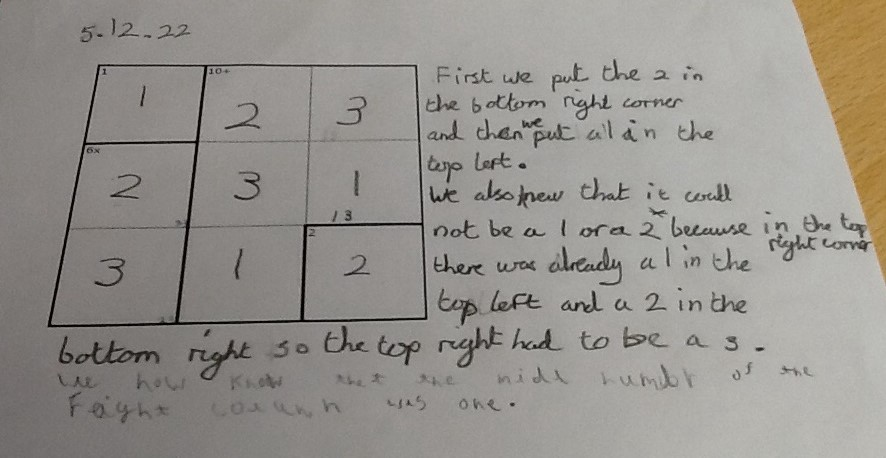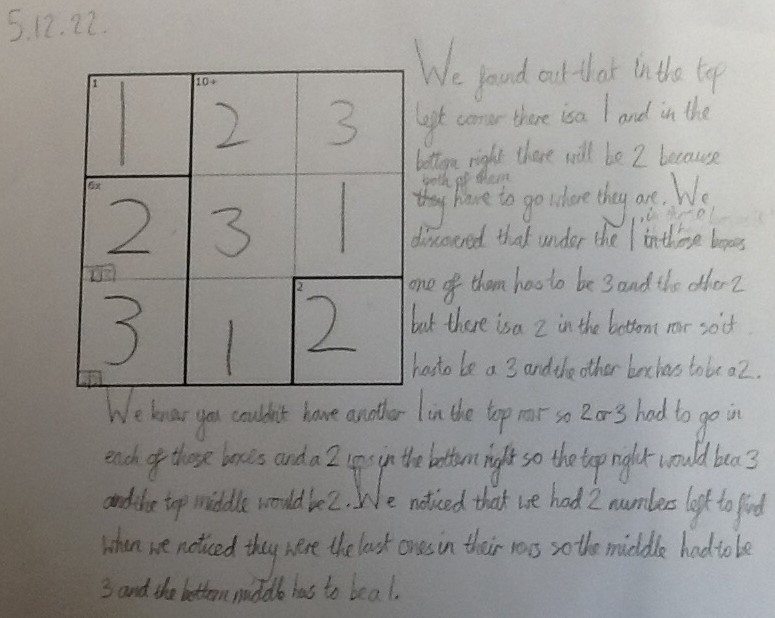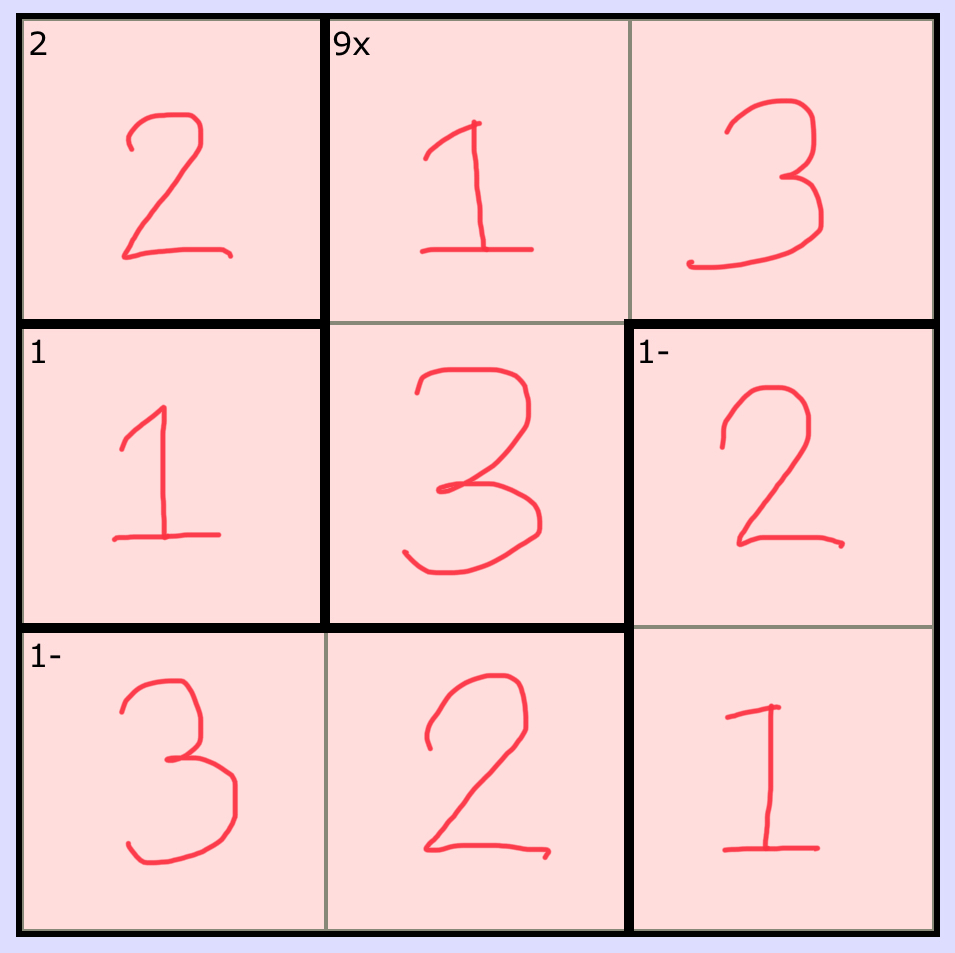#### You may also like### Pebbles

Place four pebbles on the sand in the form of a square. Keep adding as few pebbles as necessary to double the area. How many extra pebbles are added each time?### It Figures

Suppose we allow ourselves to use three numbers less than 10 and multiply them together. How many different products can you find? How do you know you've got them all?### Bracelets

Investigate the different shaped bracelets you could make from 18 different spherical beads. How do they compare if you use 24 beads?

# 3 by 3 Mathdokus

##### Age 7 to 14Challenge Level

Thank you to everybody who sent in their solutions to this activity.

We received a couple of solutions from children in Dry Drayton, England. You can click on the pictures to see the full-size solutions.

Annabel and Alice sent in this explanation of how they solved one of the puzzles:Michael and Harry sent in this step-by-step explanation of how they solved the same Mathdoku:Perri-Jane at Halstead Prep School in England explained how they solved one of the Mathdokus. They use the letter x to represent an unknown number:

This is how I worked out this 3x3 mathdoku:
Firstly, I filled in the numbers that are already given to me, 1 and 2.
Since they are in the same column, I can deduce that the bottom left corner box would be 3.
Now that I know that is 3, I have to minus 1, which is 2. So, the only number left would be 1.
Next, I have to find the value of x if x-1=1. This would leave the answer of 2. That means the only number left is 3.
Now I have 2 blank spaces, one in the middle and one in the top row, middle. I can deduce that the middle one is 3 as it is the only number left for that row and 1 for the top one, following the same procedure.
Now I know that the top row is 2,1 and 3. The middle row is 1,3 and 2. The bottom row is 3,2 and 1.Thank you all for sharing your methods with us!

We would love to hear from more of you, particularly about any strategies you used when you were stuck. Please email us if you have a solution you'd like to share with us.